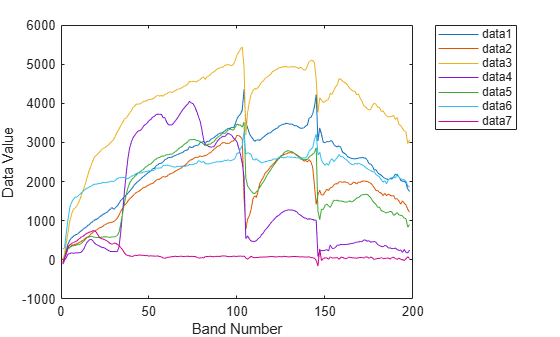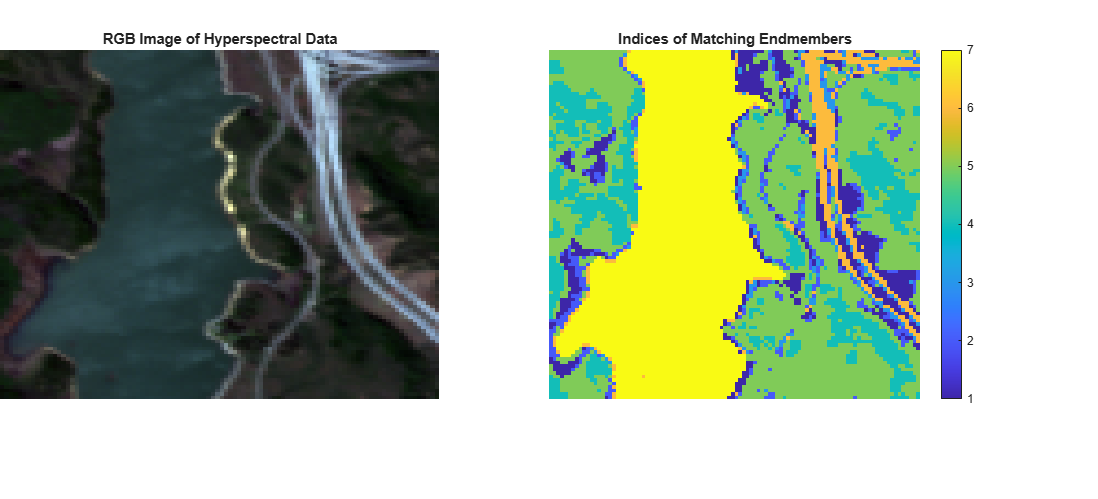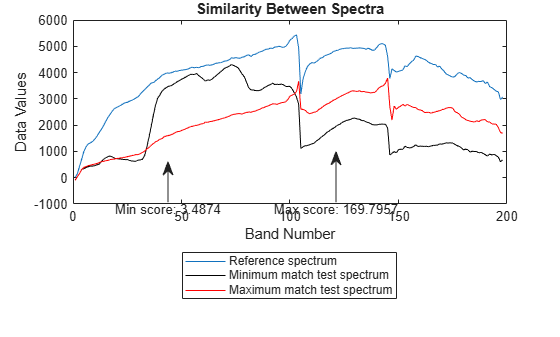sid

Measure spectral similarity using spectral information divergence

Description

example

score = sid(inputData,refSpectra) measures the spectral similarity between the spectra of each pixel in the hyperspectral data inputData and the specified reference spectra refSpectra by using the spectral information divergence (SID) technique. Use this syntax to identify different regions or materials in a hyperspectral data cube.

example

score = sid(testSpectra,refSpectra) measures the spectral similarity between the specified test spectra testSpectra and reference spectra refSpectra by using the SID method. Use this syntax to compare the spectral signature of an unknown material against the reference spectra or to compute spectral variability between two spectral signatures.

Note

This function requires the Image Processing Toolbox™ Hyperspectral Imaging Library. You can install the Image Processing Toolbox Hyperspectral Imaging Library from Add-On Explorer. For more information about installing add-ons, see Get and Manage Add-Ons.

Examples

collapse all

Distinguish different regions in a hyperspectral data cube by computing the spectral information divergence (SID) between each pixel spectrum and the endmember spectrum of the data cube.

Read hyperspectral data into the workspace.

hcube = hypercube('jasperRidge2_R198.hdr');

Specify the number of spectrally distinct bands to identify in the data cube.

numEndmembers = 7;

Extract endmember spectral signatures from the data cube by using the NFINDR algorithm.

endmembers = nfindr(hcube,numEndmembers);

Plot the spectral signatures of the endmembers.

figure
plot(endmembers)
xlabel('Band Number')
ylabel('Data Value')
legend('Location','Bestoutside')Compute the spectral information divergence between each endmember and the spectrum of each pixel in the data cube.

score = zeros(size(hcube.DataCube,1),size(hcube.DataCube,2),numEndmembers);
for i= 1:numEndmembers
score(:,:,i) = sid(hcube,endmembers(:,i));
end

Compute the minimum score value from the distance scores obtained for each pixel spectrum with respect to all the endmembers. The index of each minimum score identifies the endmember spectrum to which a pixel spectrum exhibits maximum similarity. An index value, n, at the spatial location (x, y) in the score matrix indicates that the spectral signature of the pixel at spatial location (x, y) in the data cube best matches the spectral signature of the nth endmember.

[~,matchingIndx] = min(score,[],3);

Estimate an RGB image of the hyperspectral data cube by using the colorize function. Display both the RGB image and the matrix of matched index values.

rgbImg = colorize(hcube,'Method','RGB');
figure('Position',[0 0 1100 500])
subplot('Position',[0 0.2 0.4 0.7])
imagesc(rgbImg)
axis off
colormap default
title('RGB Image of Hyperspectral Data')
subplot('Position',[0.5 0.2 0.4 0.7])
imagesc(matchingIndx);
axis off
title('Indices of Matching Endmembers')
colorbarRead hyperspectral data into the workspace.

hcube = hypercube('jasperRidge2_R198.hdr');

Find 10 endmembers of the hyperspectral data cube by using the N-FINDR method.

numEndmembers = 10;
endmembers = nfindr(hcube,numEndmembers);

Consider the first endmember as the reference spectrum and the rest of the endmembers as the test spectrum. Compute the SID score between the reference and test spectra.

score = zeros(1,numEndmembers-1);
refSpectrum = endmembers(:,1);
for i = 2:numEndmembers
testSpectrum = endmembers(:,i);
score(i-1) = sid(testSpectrum,refSpectrum);
end

Find the test spectrum that exhibit maximum similarity (minimum distance) to the reference spectrum. Then find the test spectrum that exhibit minimum similarity (maximum distance) to the reference spectrum.

[minval,minidx] = min(score);
maxMatch = endmembers(:,minidx);
[maxval,maxidx] = max(score);
minMatch = endmembers(:,maxidx);

Plot the reference spectrum, maximum similarity test spectrum, and the minimum similarity test spectrum. The test spectrum with the minimum score has the highest similarity to the reference endmember. On the other hand, the test spectrum with maximum score has the highest spectral variability and characterises the spectral behaviour of two different materials.

figure
plot(refSpectrum)
hold on
plot(maxMatch,'k')
plot(minMatch,'r')
legend('Reference spectrum','Minimum match test spectrum','Maximum match test spectrum',...
'Location','Southoutside');
title('Similarity Between Spectra')
annotation('textarrow',[0.3 0.3],[0.4 0.52],'String',['Min score: ' num2str(minval)])
annotation('textarrow',[0.6 0.6],[0.4 0.55],'String',['Max score: ' num2str(maxval)])
xlabel('Band Number')
ylabel('Data Values')Input Arguments

collapse all

Input hyperspectral data, specified as a hypercube object or a 3-D numeric array containing the data cube. If the input is a hypercube object, the data is read from the DataCube property of the object.

Test spectra, specified as a C-element vector. The test spectra is the spectral signature of an unknown region or material.

Data Types: single | double | int8 | int16 | int32 | int64 | uint8 | uint16 | uint32 | uint64

Reference spectra, specified as a C-element vector. The reference spectra is the spectral signature of a known region or material. The function matches the test spectra against these values.

Data Types: single | double | int8 | int16 | int32 | int64 | uint8 | uint16 | uint32 | uint64

Output Arguments

collapse all

SID score, returned as a scalar or matrix. The output is a

• scalar — If you specify the testSpectra input argument. The function matches the test spectral signature against the reference spectral signature and returns a scalar value. Both the test and the reference spectra must be vectors of same length.

• matrix — If you specify the inputData input argument. The function matches the spectral signature of each pixel in the data cube against the reference spectral signature and returns a matrix. If the data cube is of size M-by-N-by-C and the reference spectra is a vector of length C, the output matrix is of size M-by-N.

A smaller SAM score indicates a strong match between the test signature and the reference signature.

Data Types: single | double

collapse all

Spectral information divergence

The spectral information divergence (SID) method computes spectral similarity based on the divergence between the probability distributions of the two spectra. Let r and t be the reference and test spectra respectively. Calculate the distribution values for the reference spectra as:

${q}_{i}=\frac{{r}_{i}}{\sum _{i=1}^{C}{r}_{i}}.$

.

Calculate the distribution values for the test spectra as:

${p}_{i}=\frac{{t}_{i}}{\sum _{i=1}^{C}{t}_{i}}.$

.

Then, compute the SID value by using the probability distributions of the reference and the test spectra:

$\text{\hspace{0.17em}}\text{\hspace{0.17em}}SID=\sum _{i=1}^{C}{p}_{i}\mathrm{log}\left(\frac{{p}_{i}}{{q}_{i}}\right)+\sum _{i=1}^{C}{q}_{i}\mathrm{log}\left(\frac{{q}_{i}}{{p}_{i}}\right).$

 Chein-I Chang. “An Information-Theoretic Approach to Spectral Variability, Similarity, and Discrimination for Hyperspectral Image Analysis.” IEEE Transactions on Information Theory 46, no. 5 (August 2000): 1927–32. https://doi.org/10.1109/18.857802.